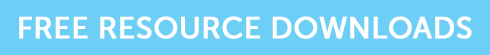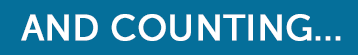A Certain Ratio – Sharpe Ratio

# A Certain Ratio – Sharpe Ratio

There is a range of measures used in the quantitative analysis of investment performance.  In this article, we look at the Sharpe ratio, named after the economist, William Sharpe.

Sharpe Ratio adjusts the rate of return of an investment to take account of its riskiness and is calculated as:

Portfolio return – Rate of return on a risk-free security / standard deviation of the return

Sharpe ratio is normally computed on an annual basis, so it is possible to compare investments on a like-with-like basis. Looking at a calculation, assume an average rate of return of 8%, a risk-free return of 3% and a standard deviation of 2.5% (remember standard deviation is a measure of the volatility of an investment or portfolio) the Sharpe ratio is:

(8 – 3) / 2.5 = 2

The Sharpe ratio adjusts portfolios for risk and basically puts them on a level risk playing field, which means they can be compared easily and equally.

A high Sharpe ratio is good, a low ratio is bad since a higher ratio indicates that the portfolio manager has made better investment decisions. A Sharpe ratio can be negative, which means the investment has underperformed risk-free assets (when someone would really have been better off in cash).

## Example

• Portfolio 1 returns 20% with a standard deviation of 10
• Portfolio 2 returns 25% with a standard deviation of 30
• The risk-free rate is 5%

Portfolio 1 = 20 – 5 / 10 = 1.5 (indicating that the portfolio earned a 1.5% return above the risk-free rate for each unit of risk taken)

Portfolio 2 = 25 – 5 / 30 = 0.67

From this example, you can see that portfolio 2 out-performed portfolio 1.  However, the Sharpe ratio tells us that portfolio 1 performed better relative to the risk in the portfolio.  If portfolio 2 had performed as well as portfolio 1 relative to its risk, it would have made a return of 50% (50 – 5 / 30) = 1.5

This ratio can be tested in all of the investment exams.  In the exam guide for J10, the question is:

A portfolio has provided annual returns over the last 3 years of 5%, 9% and 10%, compared to a return over the same period from a portfolio of treasury bills, of 4% per annum.  If the standard deviation of the portfolio is 10%, the Sharpe ratio for the portfolio over 3 years is:

1. 0.4
2. 0.5
3. 0.6
4. 0.8

With this question, work out the ratio for each year, and divide the answer by 3 – the answer is A.

## Grab the resources you need!

If you’re studying for your CII AF4 exam, and you’re wanting some extra practice, grab our free taster to try out one of Brand Financial Training’s resources for yourself.  Click the link to download the AF4 mock paper taster now!Alternatively, you can download the mock paper taster for J10J12 or R02 one of those exams is stressing you out.